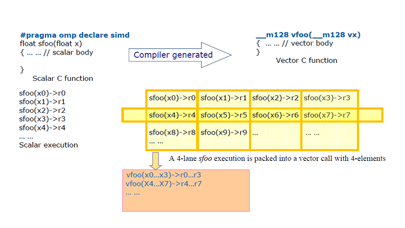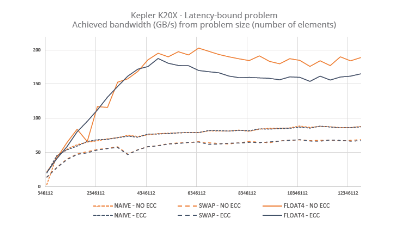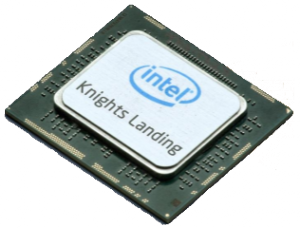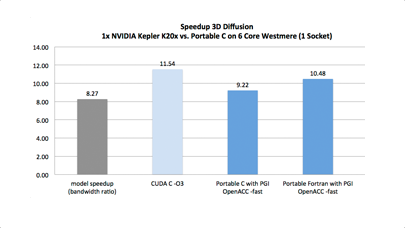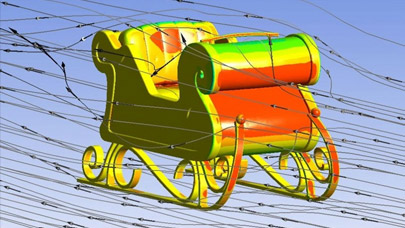Explicit Vector Programming with OpenMP 4.0 SIMD Extensions
By and   |  November 19, 2014

3 – SIMD PROGRAMMING GUIDELINE

This section provides several programming guidelines for OpenMP programmers to develop correct and high performance SIMD programs using SIMD extensions in the OpenMP 4.0 specification.

3.1 – Ensure SIMD execution legality

If programmers apply the SIMD construct to loops so that they are transformed into SIMD loops, they guarantee that the loops can be partitioned into chunks such that iterations within each chunk can correctly execute concurrently using SIMD instructions. To provide this guarantee, the programmers must use the `safelen` clause to preserve all original data dependencies or remove data dependencies that prevent SIMD execution by specifying data sharing clauses such as `private`, `lastprivate` or `reduction`. Recall that:

• A loop annotated with a SIMD pragma/directive has logical iterations numbered 0,1,…,N-1 where N is the number of loop iterations;

• The logical numbering denotes the sequence in which the iterations would execute if the associated loop(s) executed with no SIMD instructions;

• If the `safelen(L)` clause is specified, then no two iterations executed concurrently with SIMD instructions can have a greater distance in the logical iteration space than L.

Programmers can use two mechanisms to ensure that an OpenMP canonical loop can legally be transformed for SIMD execution.

Mechanism 1: Given a logical loop iteration, use the `safelen` clause to prohibit loop-carried lexically backward dependencies between any two iterations in the chunk. For example, if the chunk is [k, k+1, k+2, k+3], then the results produced by the iteration k must not be consumed by the iteration k+1, k+2 and k+3.

```<strong>#pragma omp simd safelen(4)</strong>
for (k=5; k<N; k++)  {
a[k] = a[k-4] + b[k];
}```

listing 3.1.1 – A code example of SIMD loop with a safelen clause.

The `safelen` in listing 3.1.1 asserts the loop may be safely SIMDized with any vector length (VL) less than 5. Assuming VL = 4 is selected by the compiler, its SIMD execution is shown below. The `iteration-n` denotes the serial execution logical iteration number, `vector iteration-n` denotes the SIMD execution logical vector iteration number, `r-n` denotes general scalar register, `vr-n` vector register.Fig. 3.1.2. - Execution samples of simd loop with safelen clause.

In Fig. 3.1.2, from the serial execution of logical iterations of the loop, the result produced by `iteration-0 store a` is carried over to `iteration-4 load a`; the result produced by `iteration-1 store a` is carried over to `iteration-5 load a`, and so on. In addition, the lexical order of `store a[k]` to `load a[k-4]` is backward; thus, this loop has a loop-carried lexically backward dependency between iteration `iteration-(k)` and `iteration-(k+4)` where k=0, 1,2,…N-4. In other words, this loop cannot be vectorized with VL>4. Since the programmer specified `safelen(4)`, the compiler can correctly vectorize the loop, as the above table shows for the SIMD execution of `vector iteration-0` and `vector iteration-1`.

Mechanism 2: Given a logical loop iteration space, use data sharing clauses to ensure that no two iterations in any chunk have `write-write` or `write-read` conflicts. The example below demonstrates how the `private` clause can be used to achieve this effect.

```{ float x; #pragma omp simd private(x) for (k = 0; k<N; k++) { x = a[k]; b[k] = foo(x+a[k+1]); } }```

listing 3.1.3 – A code example of SIMD loop with a private clause.

In listing 3.1.3, the op may be safely vectorized with any `VL < N` by privatizing the variable `x`. However, if `x` is not privatized for each SIMD lane, there will be a write-write and write-read conflict involving `x`.

3.2 Effective use of the `uniform` and `linear` clauses

The `uniform` clause directs the compiler to generate code that passes the parameter’s value (or address if it is a pointer) via a scalar register instead of a vector register. The `linear` clause for a scalar parameter (or variable) directs the compiler to generate code that loads/stores its value using a scalar register instead of a vector register. For a pointer, the clause directs the compiler to generate a unit-stride (or the explicitly indicated stride in `linear-step`) load/store vector instruction (e.g., `movups`) to load/store data to a vector register.

A typical case is that the base address of a memory access is `uniform`, and the index (or offset) has the `linear` property. Putting them together, the compiler generates linear unit-stride memory load/store instructions to obtain performance gains. Given the example below, the function `SqrtMul` is marked with `omp declare simd` annotation:

```<strong>#pragma omp declare simd uniform(op1) linear(k) notinbranch</strong>
double SqrtMul(double *op1, double op2, int k) {
return (sqrt(op1[k]) * sqrt(op2));
}```

listing 3.2.1 – A code example of SIMD loop with uniform and linear clauses.

Given the example in listing 3.2.1, the compiler generates the following vector `SqrtMul` function on the Core i7 processor, for the faster SIMD vector function. The `uniform(op1)` and `linear(k:1)` attributes allow the compiler to pass the base address in the eax register and the initial offset value in the ecx register for the 32-bit memory address computation, and then, use one `movups` instruction to load two 64-bit floating-point data (`a[k]` and `a[k+1]`) to a 128-bit XMM register, as shown in Figure 3.2.2.

```;;  Generated vector function for
;;  #pragma omp declare simd uniform(op1) linear(k:1) notinbranch
PUBLIC _ZGVxN4uvl_SqrtMul.P
; parameter 1: eax    ; uniform op1
; parameter 2: xmm0   ; xmm0 holds op2_1 and op2_2
; parameter 3: ecx    ; linear k with unit stride
movups  xmm1, XMMWORD PTR [eax+ecx*8]
; xmm1 holds op1[k] and op1[k+1]
sqrtpd  xmm0, xmm0 ; vector_sqrt(xmm0)
sqrtpd  xmm2, xmm1 ; vector_sqrt(xmm1)
mulpd   xmm0, xmm2 ; vector multiply
ret```

listing 3.2.2 – SSE4.2 code generated for the SIMD function with uniform and linear clauses.

If the programmer omits the `uniform` and `linear` clauses, the compiler cannot determine that the memory loads /stores of all SIMD lanes have the same base address and that their offset is a linear unit-stride. Thus, the compiler must use XMM registers for passing `op1`, `op2` and `k` for the scalar function `SqrtMul` under a SIMD execution context, as the example shows in listing 3.2.3. For the memory address computation, the compiler generates memory load instruction `movq` for loading `op1_1[k1]` to xmm3 register low quadword and `movhpd` for loading `op1_2[k2]` to xmm3 register high quadword in order to perform vector execution of `vector_sqrt(xmm3)` using the vector instruction `sqrtpd`. Calling this version provides much lower performance, even if the function invocation at call site passes in a uniform memory address `op1` and a linear unit-stride value `k`.

```;;  Generated vector function for #pragma omp declare simd notinbranch
PUBLIC _ZGVxN4vvv_SqrtMul.P
; parameter 1: xmm0    ; vector_op1 holds op1_1 and op1_2
; parameter 2: xmm1    ; vector_op2 holds op2_1 and op2_2
; parameter 3: xmm2    ; vector_k   holds k1 and k2
pslld     xmm2, 3      ; vector_k*8 is index value
paddd     xmm0, xmm2       ; vector_op1 + vector_k*8
movd      eax,  xmm0       ; load op1_1 + k1*8 to EAX
pshuflw   xmm2, xmm0, 238  ; shift to get opt1_2 + k2*8
movd      edx,  xmm2       ; load op1_2 + k2*8 to EDX
sqrtpd    xmm0, xmm1       ; vector_sqrt(xmm1)
movq      xmm3, QWORD PTR [eax] ; load op1_1[k1] to xmm3 low quadword
movhpd    xmm3, QWORD PTR [edx] ; load op1_2[k2] to xmm3 high quadword
sqrtpd    xmm3, xmm3       ; vector_sqrt(xmm3)
mulpd     xmm0, xmm3       ; vector multiply
ret```

listing 3.2.3 – SSE4.2 code generated for the SIMD function without uniform and linear clauses.

The vector variant function generated from the small kernel program in listing 3.2.4 that uses the `uniform` and `linear` clauses produces a 1.62x speedup over one that omits the clauses, when it is compiled with options -xSSE4.2 and -DSIMDOPT using the Intel C++ compiler. The generated SIMD vector executable runs on an Intel Core i7 processor with a 64-bit Linux OS installed.

```#include <stdio.h>
#include <stdlib.h>
#define M 1000000
#define N 1024
</code><code>
void init(float a[]) {
int i;
for (i = 0; i < N; i++) a[i] = (float)i*1.5;
}
</code><code>
float checksum(float b[]) {
int i;
float res = 0.0;
for (i = 0; i < N; i++) res += b[i]; return res;
}
```
```
<strong>#pragma omp declare simd simdlen(8)
#ifdef SIMDOPT
#pragma omp declare simd linear(op1) uniform(op2) simdlen(8)
#endif</strong>
float fSqrtMul(float *op1, float op2) {
return sqrt(*op1)*sqrt(op2);
}
```
```int main(int argc, char *argv[]) {
int i, k; float a[N], b[N];
float res = 0.0f; init(a);
for (i = 0; i < M; i++) {
float op2 = 64.0f + i*1.0f;
<strong>#pragma omp simd</strong>
for (k=0; k<N; k++) {
b[k] = fSqrtMul(&a[k], op2);
}
}
res = checksum(b); printf("res = %.2fn", res);
}```

listing 3.2.4 - An example using uniform and linear clauses.

<12345678>

More around this topic...

In the same sectionBy the same authorThank you for reading HPC Today.

Express poll

Do you use multi-screen
visualization technologies?

Industry news

Trending this week...